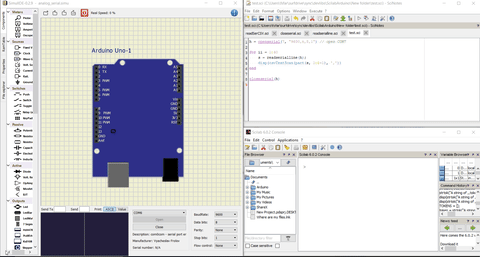# Data acquisition : From sensor, to SciLAB, through serial port

Hello everybody,

I want to plot data given by a capacitive sensor made with CapSense library in SciLAB.

The CapSense code allow the user to print on the serial port a value that is proportional to capacitance.

On the other side, there's a function in "Serial port communication" library of SciLAB that read the data in the serial port and extract a string of it.

So it is possible to print the capacitive sensor value in the serial port terminal and read it immediately with SciLAB in order to convert the string in SciLAB in float and store the value in a vector for plot it.

The problem is :

Values of capacitive sensor are printed every 100ms in the serial port terminal and whatever the way they are printed (524545 or 45 56 65 or 45,87,98,120), this is a difficult work to extract the correct string of the monitor.

So, is there a way to print in the serial monitor the values ONE by ONE, every 100ms ? In order to make the "extraction" of the data in SciLAB easier ?

Thanks a lot

Post the Arduino code, using code tags.

Thanks for being here and for responding at such short notice.

The code is the following :

``````#include <CapacitiveSensor.h>

CapacitiveSensor   cs_4_2 = CapacitiveSensor(4,2);        // 10M resistor between pins 4 & 2, pin 2 is sensor pin, add a wire and or foil if desired

void setup()
{
cs_4_2.set_CS_AutocaL_Millis(0xFFFFFFFF);     // turn off autocalibrate on channel 1 - just as an example
Serial.begin(9600);
}

void loop()
{
long start = millis();
long total1 =  cs_4_2.capacitiveSensor(30);

Serial.print(total1);                  // print sensor output 1

delay(100);                             // arbitrary delay to limit data to serial port
}
``````

This is the basic code written by Paul Badger for a capacitive sensor in CapSense Library.

In SciLAB, I use the "Serial port communication" library that contain the following functions :

h = openserial(5,"9600");

closeserial;

Here I open the port COM5 and I read 3 characters in the serial monitor. "data" is a string that I convert in float to store it in an array for plotting.

The problem is that currently in te serial monitor we can read the values the ones next to the others and when I use serialread function in SciLAB I have no control on what is read.

For example if I have 3 consecutives values like 100,200,300, in the serial monitor is printed :

100200300

and if I use the SciLAB function, the result can be:

data=300 -> ok

or

data=030 -> WRONG.

Do you have an idea ? How can I fix this ?

Thank you

The Arduino IS printing out the values one at a time.
Your problem is a Scilab problem. Reading three characters is not the solution, but I'm not a Scilab expert. You probably need to recognize CR or LF characters.

Ok thanks, I will search an answer in SciLAB. It's a good idea to check the reading of CR character !

If I have the solution, I will post it and mark the topic as "Solved" because I think this would be usefull for other users.

Plotting data from serial port is quite interesting.

Have a good day

Try this.

Serial.print ("\n");

if yout first 3 values are 100,200,300
You will print them this way:

100
200
300

Hope this helps,
Regards,

IMT Carlos Gongora

First of all Aditya Sengupta hasn't updated his repository for the last 8 years and there have been already a lot of issues reported everywhere. Unfortunately the pull requests and issue reports have also been around for a long time. So I decided to fork the repo with many improvements here:

but to answer your question, you can write your information in CSV format into the serial port and then use the csvTextScan to convert them into matrix. I have explained everything here in details. and a demonstration can also be found here with instructions:P.S. It turns out that I rewrote the tool box from scratch so it has the similar functionality as the MATLAB toolbox. You may find the latest prototype hereand a demo here.